# Tangents

## Calculus 1 / Ab### 11 Practice Problems

04:24Thomas Calculus 12

The accompanying figure shows the plot of distance fallen versus time for an object that fell from the lunar landing module a distance 80 $\mathrm{m}$ to the surface of the moon.
a. Estimate the slopes of the secants $P Q_{1}, P Q_{2}, P Q_{3},$ and $P Q_{4}$ arranging them in a table like the one in Figure 2.6 .
b. About how fast was the object going when it hit the surface?

Limits and Continuity
Rates of Change and Tangents to Curves02:23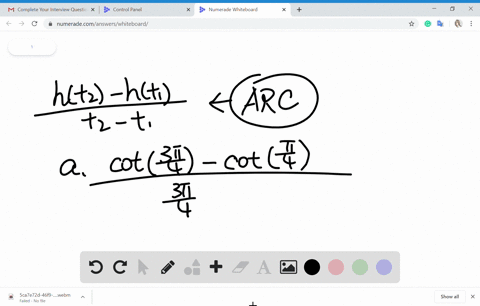Thomas Calculus 12

Find the average rate of change of the function over the given interval or intervals.
$$h(t)=\cot t$$
$$\text { a. }[\pi / 4,3 \pi / 4] \quad \text { b. }[\pi / 6, \pi / 2]$$

Limits and Continuity
Rates of Change and Tangents to Curves08:11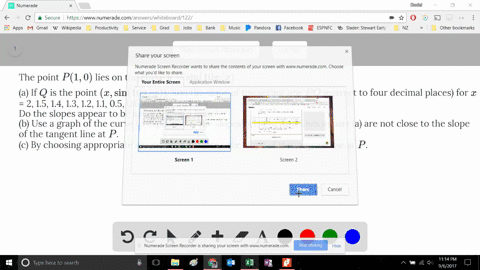Calculus: Early Transcendentals

The point $P(1, 0)$ lies on the curve $y = \sin (10\pi /x)$.

(a) If $Q$ is the point $(x, \sin (10\pi /x))$, find the slope of the secant line $PQ$ (correct to four decimal places) for $x$ = 2, 1.5, 1.4, 1.3, 1.2, 1.1, 0.5, 0.6, 0.7, 0.8, and 0.9 .
Do the slopes appear to be approaching a limit?
(b) Use a graph of the curve to explain why the slopes of the secant lines in part (a) are not close to the slope of the tangent line at $P$.
(c) By choosing appropriate secant lines, estimate the slope of the tangent line at $P$.

Limits and Derivatives
The Tangent and Velocity Problems04:53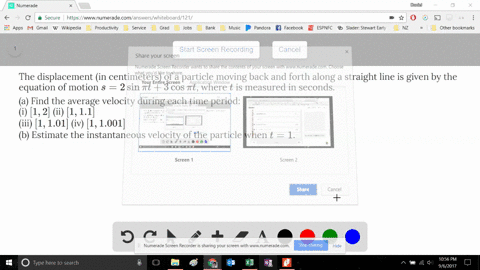Calculus: Early Transcendentals

The displacement (in centimeters) of a particle moving back and forth along a straight line is given by the equation of motion $s = 2 \sin \pi t + 3 \cos \pi t$, where $t$ is measured in seconds.

(a) Find the average velocity during each time period:
(i) $[1, 2]$ (ii) $[1, 1.1]$
(iii) $[1, 1.01]$ (iv) $[1, 1.001]$
(b) Estimate the instantaneous velocity of the particle when $t =1$.

Limits and Derivatives
The Tangent and Velocity Problems05:46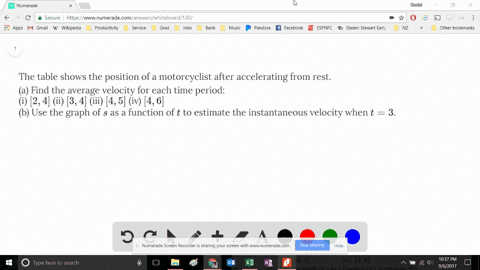Calculus: Early Transcendentals

The table shows the position of a motorcyclist after accelerating from rest.

(a) Find the average velocity for each time period:
(i) $[2, 4]$ (ii) $[3, 4]$ (iii) $[4, 5]$ (iv) $[4, 6]$
(b) Use the graph of $s$ as a function of $t$ to estimate the instantaneous velocity when $t = 3$.

Limits and Derivatives
The Tangent and Velocity Problems05:25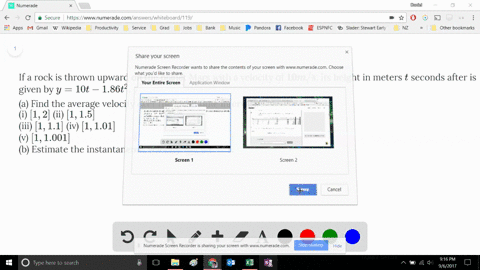Calculus: Early Transcendentals

If a rock is thrown upward on the planet Mars with a velocity of $10 m/s$, its height in meters $t$ seconds after is given by $y = 10t - 1.86t^2$.

(a) Find the average velocity over the given time intervals:
(i) $[1, 2]$ (ii) $[1, 1.5]$
(iii) $[1, 1.1]$ (iv) $[1, 1.01]$
(v) $[1, 1.001]$
(b) Estimate the instantaneous velocity when $t = 1$.

Limits and Derivatives
The Tangent and Velocity Problems05:24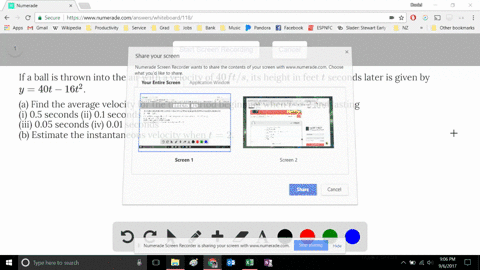Calculus: Early Transcendentals

If a ball is thrown into the air with a velocity of $40 ft/s$, its height in feet $t$ seconds later is given by $y = 40t - 16t^2$.

(a) Find the average velocity for the time period beginning when $t = 2$ and lasting
(i) 0.5 seconds (ii) 0.1 seconds
(iii) 0.05 seconds (iv) 0.01 seconds
(b) Estimate the instantaneous velocity when $t = 2$.

Limits and Derivatives
The Tangent and Velocity Problems07:22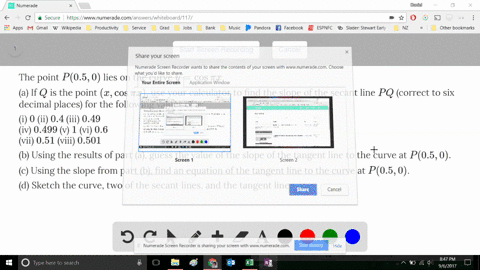Calculus: Early Transcendentals

The point $P(0.5, 0)$ lies on the curve $y = \cos \pi x$.

(a) If $Q$ is the point $(x, \cos \pi x)$, use your calculator to find the slope of the secant line $PQ$ (correct to six decimal places) for the following values of $x$:

(i) $0$ (ii) $0.4$ (iii) $0.49$
(iv) $0.499$ (v) $1$ (vi) $0.6$
(vii) $0. 51$ (viii) $0.501$

(b) Using the results of part (a), guess the value of the slope of the tangent line to the curve at $P(0.5, 0)$.

(c) Using the slope from part (b), find an equation of the tangent line to the curve at $P(0.5, 0)$.

(d) Sketch the curve, two of the secant lines, and the tangent line.

Limits and Derivatives
The Tangent and Velocity Problems06:22Calculus: Early Transcendentals

The point $P(2, -1)$ lies on the curve $y = 1/(1-x)$.

(a) If $Q$ is the point $(x, 1/(1-x))$, use your calculator to find the slope of the secant line $PQ$ (correct to six decimal places) for the following values of $x$:
(i) $1.5$ (ii) $1.9$ (iii) $1.99$ (iv) $1.999$
(v) $2.5$ (vi) $2.1$ (vii) $2.01$ (viii) $2.001$

(b) Using the results of part (a), guess the value of the slope of the tangent line to the curve at $P(2, -1)$.

(c) Using the slope from part (b), find an equation of the tangent line to the curve at $P(2, -1)$.

Limits and Derivatives
The Tangent and Velocity Problems04:40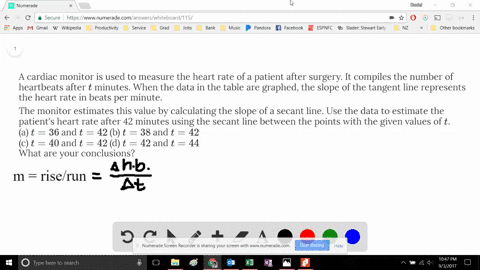Calculus: Early Transcendentals

A cardiac monitor is used to measure the heart rate of a patient after surgery. It compiles the number of heartbeats after $t$ minutes. When the data in the table are graphed, the slope of the tangent line represents the heart rate in beats per minute.

The monitor estimates this value by calculating the slope of a secant line. Use the data to estimate the patient's heart rate after 42 minutes using the secant line between the points with the given values of $t$.
(a) $t = 36$ and $t = 42$ (b) $t = 38$ and $t = 42$
(c) $t = 40$ and $t = 42$ (d) $t = 42$ and $t = 44$

Limits and Derivatives
The Tangent and Velocity Problems06:40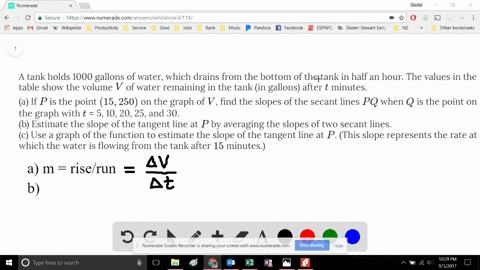Calculus: Early Transcendentals

A tank holds 1000 gallons of water, which drains from the bottom of the tank in half an hour. The values in the table show the volume $V$ of water remaining in the tank (in gallons) after $t$ minutes.

(a) If $P$ is the point $(15, 250)$ on the graph of $V$, find the slopes of the secant lines $PQ$ when $Q$ is the point on the graph with $t$ = 5, 10, 20, 25, and 30.
(b) Estimate the slope of the tangent line at $P$ by averaging the slopes of two secant lines.
(c) Use a graph of the function to estimate the slope of the tangent line at $P$. (This slope represents the rate at which the water is flowing from the tank after $15$ minutes.)

Limits and Derivatives
The Tangent and Velocity Problems1